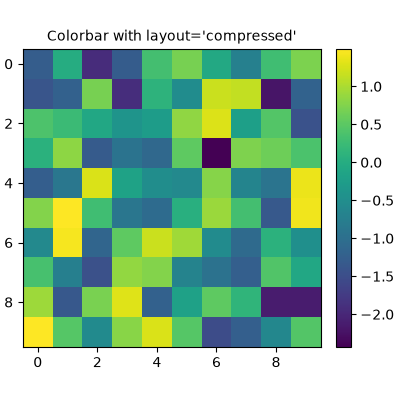# Placing Colorbars#

Colorbars indicate the quantitative extent of image data. Placing in a figure is non-trivial because room needs to be made for them.

## Automatic placement of colorbars#

The simplest case is just attaching a colorbar to each axes. Note in this example that the colorbars steal some space from the parent axes.

```import matplotlib.pyplot as plt
import numpy as np

# Fixing random state for reproducibility
np.random.seed(19680801)

fig, axs = plt.subplots(2, 2)
cmaps = ['RdBu_r', 'viridis']
for col in range(2):
for row in range(2):
ax = axs[row, col]
pcm = ax.pcolormesh(np.random.random((20, 20)) * (col + 1),
cmap=cmaps[col])
fig.colorbar(pcm, ax=ax)
```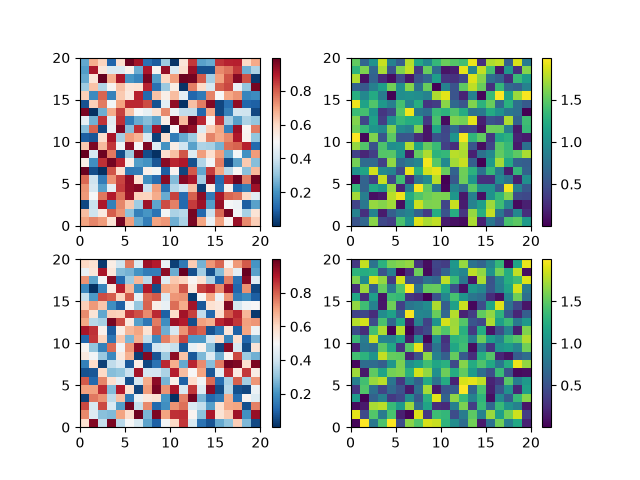The first column has the same type of data in both rows, so it may be desirable to have just one colorbar. We do this by passing `Figure.colorbar` a list of axes with the ax kwarg.

```fig, axs = plt.subplots(2, 2)
cmaps = ['RdBu_r', 'viridis']
for col in range(2):
for row in range(2):
ax = axs[row, col]
pcm = ax.pcolormesh(np.random.random((20, 20)) * (col + 1),
cmap=cmaps[col])
fig.colorbar(pcm, ax=axs[:, col], shrink=0.6)
```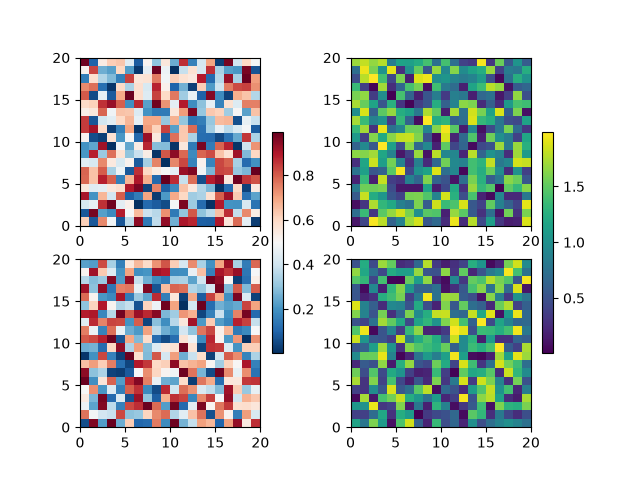The stolen space can lead to axes in the same subplot layout being different sizes, which is often undesired if the the x-axis on each plot is meant to be comparable as in the following:

```fig, axs = plt.subplots(2, 1, figsize=(4, 5), sharex=True)
X = np.random.randn(20, 20)
axs.plot(np.sum(X, axis=0))
axs.pcolormesh(X)
fig.colorbar(pcm, ax=axs, shrink=0.6)
```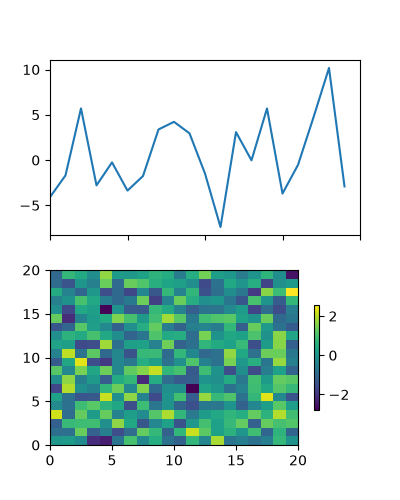This is usually undesired, and can be worked around in various ways, e.g. adding a colorbar to the other axes and then removing it. However, the most straightforward is to use constrained layout:

```fig, axs = plt.subplots(2, 1, figsize=(4, 5), sharex=True, layout='constrained')
axs.plot(np.sum(X, axis=0))
axs.pcolormesh(X)
fig.colorbar(pcm, ax=axs, shrink=0.6)
```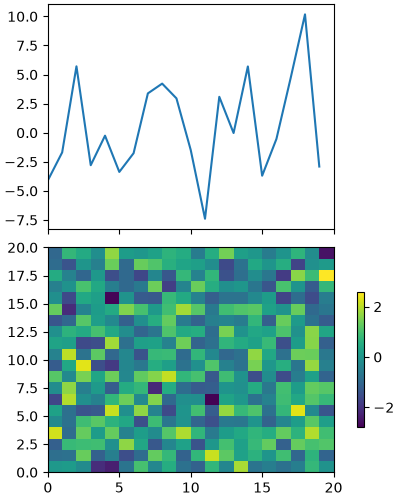Relatively complicated colorbar layouts are possible using this paradigm. Note that this example works far better with `layout='constrained'`

```fig, axs = plt.subplots(3, 3, layout='constrained')
for ax in axs.flat:
pcm = ax.pcolormesh(np.random.random((20, 20)))

fig.colorbar(pcm, ax=axs[0, :2], shrink=0.6, location='bottom')
fig.colorbar(pcm, ax=[axs[0, 2]], location='bottom')
fig.colorbar(pcm, ax=axs[1:, :], location='right', shrink=0.6)
fig.colorbar(pcm, ax=[axs[2, 1]], location='left')
```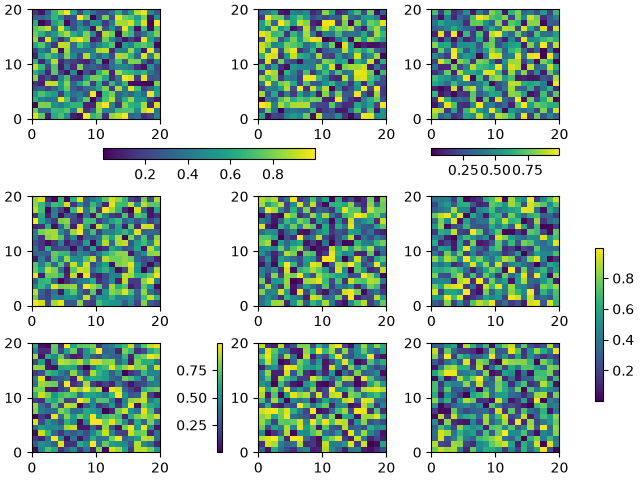## Adjusting the spacing between colorbars and parent axes#

The distance a colorbar is from the parent axes can be adjusted with the pad keyword argument. This is in units of fraction of the parent axes width, and the default for a vertical axes is 0.05 (or 0.15 for a horizontal axes).

```fig, axs = plt.subplots(3, 1, layout='constrained', figsize=(5, 5))
for ax, pad in zip(axs, [0.025, 0.05, 0.1]):
pcm = ax.pcolormesh(np.random.randn(20, 20), cmap='viridis')
fig.suptitle("layout='constrained'")
```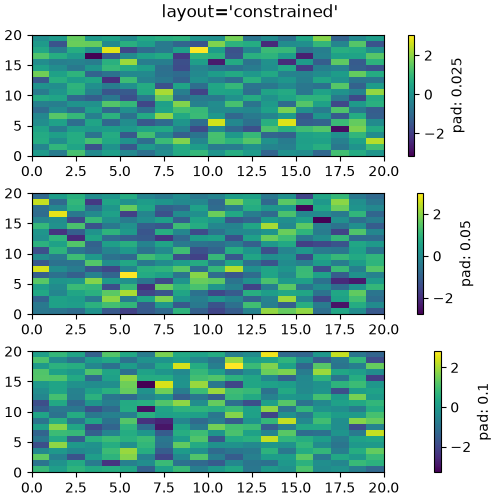Note that if you do not use constrained layout, the pad command makes the parent axes shrink:

```fig, axs = plt.subplots(3, 1, figsize=(5, 5))
for ax, pad in zip(axs, [0.025, 0.05, 0.1]):
pcm = ax.pcolormesh(np.random.randn(20, 20), cmap='viridis')
fig.suptitle("No layout manager")
```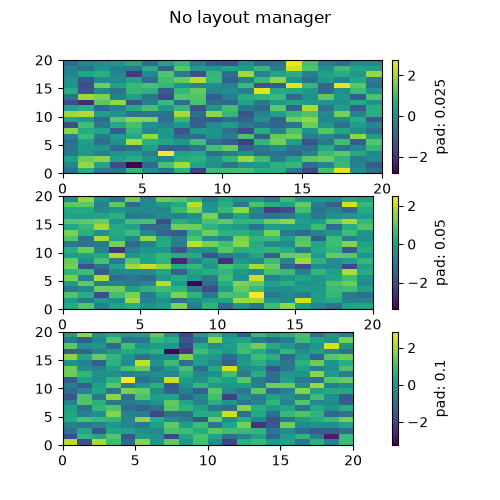## Manual placement of colorbars#

Sometimes the automatic placement provided by `colorbar` does not give the desired effect. We can manually create an axes and tell `colorbar` to use that axes by passing the axes to the cax keyword argument.

### Using `inset_axes`#

We can manually create any type of axes for the colorbar to use, but an `Axes.inset_axes` is useful because it is a child of the parent axes and can be positioned relative to the parent. Here we add a colorbar centered near the bottom of the parent axes.

```fig, ax = plt.subplots(layout='constrained', figsize=(4, 4))
pcm = ax.pcolormesh(np.random.randn(20, 20), cmap='viridis')
ax.set_ylim([-4, 20])
cax = ax.inset_axes([0.3, 0.07, 0.4, 0.04])
fig.colorbar(pcm, cax=cax, orientation='horizontal')
```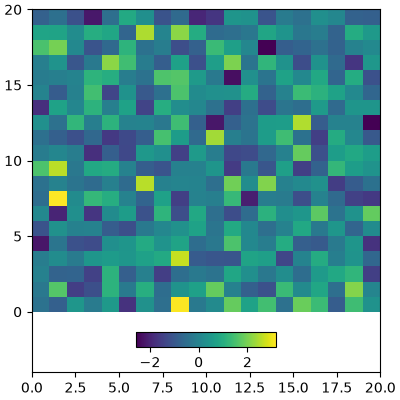`Axes.inset_axes` can also specify its position in data coordinates using the transform keyword argument if you want your axes at a certain data position on the graph:

```fig, ax = plt.subplots(layout='constrained', figsize=(4, 4))
pcm = ax.pcolormesh(np.random.randn(20, 20), cmap='viridis')
ax.set_ylim([-4, 20])
cax = ax.inset_axes([7.5, -1.7, 5, 1.2], transform=ax.transData)
fig.colorbar(pcm, cax=cax, orientation='horizontal')
```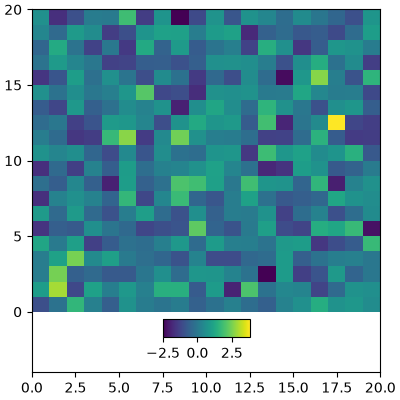### Colorbars attached to fixed-aspect-ratio axes#

Placing colorbars for axes with a fixed aspect ratio pose a particular challenge as the parent axes changes size depending on the data view.

```fig, axs = plt.subplots(2, 2,  layout='constrained')
cmaps = ['RdBu_r', 'viridis']
for col in range(2):
for row in range(2):
ax = axs[row, col]
pcm = ax.pcolormesh(np.random.random((20, 20)) * (col + 1),
cmap=cmaps[col])
if col == 0:
ax.set_aspect(2)
else:
ax.set_aspect(1/2)
if row == 1:
fig.colorbar(pcm, ax=ax, shrink=0.6)
```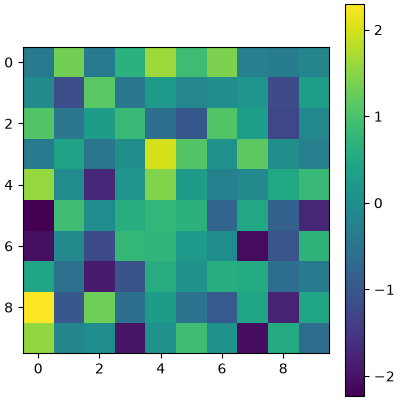We solve this problem using `Axes.inset_axes` to locate the axes in "axes coordinates" (see Transformations Tutorial). Note that if you zoom in on the parent axes, and thus change the shape of it, the colorbar will also change position.

```fig, axs = plt.subplots(2, 2, layout='constrained')
cmaps = ['RdBu_r', 'viridis']
for col in range(2):
for row in range(2):
ax = axs[row, col]
pcm = ax.pcolormesh(np.random.random((20, 20)) * (col + 1),
cmap=cmaps[col])
if col == 0:
ax.set_aspect(2)
else:
ax.set_aspect(1/2)
if row == 1:
cax = ax.inset_axes([1.04, 0.2, 0.05, 0.6])
fig.colorbar(pcm, cax=cax)
```